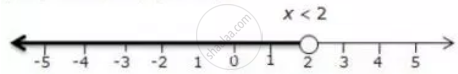Share

Represent the Solution of the Given Inequalities on the Real Number Line (2x + 5)/3 > 3x - 3 - ICSE Class 10 - Mathematics

ConceptLinear Inequations in One Unknown

Question

Represent the solution of the given inequalities on the real number line

(2x + 5)/3 > 3x - 3

Solution

(2x + 5)/3 > 3x - 3

2x + 5 > 9x - 9

-7x > - 14

x < 2

The solution on number line is:Is there an error in this question or solution?

APPEARS IN

Solution Represent the Solution of the Given Inequalities on the Real Number Line (2x + 5)/3 > 3x - 3 Concept: Linear Inequations in One Unknown.
S